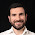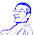## Tuesday, September 25, 2018

### PCA plot with fill, color, and shape all together

When I plotted the PCA results (e.g. scatter plot for PC1 and PC2) and was about to annotate the dataset with different covariates (e.g. gender, diagnosis, and ethic group), I noticed that it's not straightforward to annotate >2 covariates at the same time using ggplot.

Here is what works for me in ggplot:

pcaData <- plotPCA(vsd, intgroup = c( "Diagnosis", "Ethnicity", "Sex"), returnData = TRUE) # vsd and plotPCA are part of DESeq2 package, nothing with my example below.
percentVar <- round(100 * attr(pcaData, "percentVar"))
ggplot(pcaData, aes(x = PC1, y = PC2, color = factor(Diagnosis), shape = factor(Ethnicity))) +
geom_point(size =3, aes(fill=factor(Diagnosis), alpha=as.character(Sex))) +
geom_point(size =3) +
scale_shape_manual(values=c(21,22)) +
scale_alpha_manual(values=c("F"=0, "M"=1)) +
xlab(paste0("PC1: ", percentVar, "% variance")) +
ylab(paste0("PC2: ", percentVar, "% variance")) +
ggtitle("PCA of all genes, no covariate adjusted")

I also found that you can use the male and female symbol (♂ ♀) as shapes in your plot. Here is how:

df <- data.frame(x = runif(10), y = runif(10), sex = sample(c("m","f"), 10, rep = T))
qplot(x, y, data = df, shape = sex, size = I(5)) +
scale_shape_manual(values = c("m" = "\u2642", f = "\u2640"))
(Reference: https://github.com/kmiddleton/rexamples/blob/master/ggplot2%20male-female%20symbols.R)

I've not figured out a way to combine the two ideas above.

1.1.